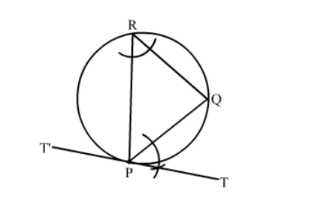# Draw a circle of radius 4.8 cm. Take a point P on it.`
Question:

Draw a circle of radius 4.8 cm. Take a point P on it. Without using the centre of the circle, construct a tangent at the point P. Write the steps of construction.

Solution:

Steps of Construction  :

Step 1. Draw a circle of radius 4.8 cm.
Step 2. Mark a point P on it.
Step 3. Draw any chord PQ.
Step 4. Take a point R on the major arc QP.
Step 5. Join PR and RQ.

Step 6. Draw $\angle Q P T=\angle P R Q$

Step 7. Produce TP to T', as shown in the figure.T'PT is the required tangent.